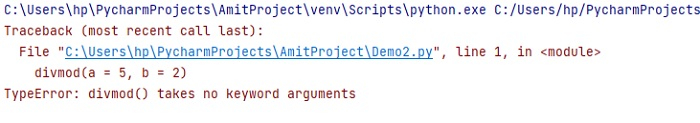# What does the slash(/) in the parameter list of a function mean in Python?

A slash in the argument list of a function denotes that the parameters prior to it are positional-only. Let us first see a function in Python with a parameter −

## Function in Python

### Example

Here, we are creating a basic function in Python with a parameter myStr −

# Creating a Function
def demo(myStr):
print("Car =: ",myStr)

# function call
demo("BMW")
demo("Tesla")


### Output

Car =: BMW
Car =: Tesla


## Slash in the parameter list of a function

As said above, a slash in the argument list of a function denotes that the parameters prior to it are positional-only.

Upon calling a function that accepts positional-only parameters, arguments are mapped to parameters based solely on their position.

## The divmode() function

The divmod() function is the perfect example of a slash in the list of a function i.e. it accepts positional-only parameters as shown below −

divmod(a, b, /)


Above, since the slash is at the end of the parameter list , both the parameters a and b are positional-only.

Let us print the documentation of divmod() using the help() functiojn in Python

# Creating a Function
def demo(myStr):
print(help(divmod))

# function call
demo("BMW")
demo("Tesla")


### Output

Help on built-in function divmod in module builtins:

divmod(x, y, /)
Return the tuple (x//y, x%y). Invariant: div*y + mod == x.
None


Now, let us see an example of the divmod(). Both the parameters are dividend and divisor −

k = divmod(5, 2)

print(k)


### Output

(2, 1)


The slash at the end of the parameter list means that both parameters are positional-only. Therefore, an error would arise, if we will call the divmod() with keyword arguments −

divmod(a = 5, b = 2)


### OutputIn the above example, an error occurred since the divmod() takes no keyword arguments.